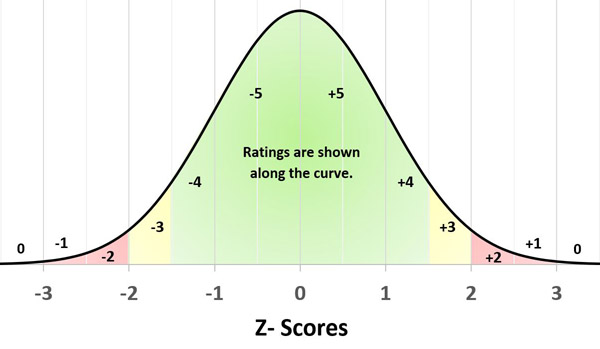New customer? Register here

 Announcements About Us AASHTO Accreditation Laboratory Assessments Proficiency Samples Falling Weight Deflectometer re:university Specifiers

The gold standard for laboratory accreditation in the construction industry

# PSP Analysis Description

## Removing Unpaired, Invalid and Outlier Data

After collecting the data, blank results and "unpaired" results are removed from the dataset. Unpaired results are characterized by a blank result for either sample X (the odd numbered sample) or sample Y (the even numbered sample). The new "paired" dataset is then subjected to a two-step routine to remove "invalid" and "outlier" values. First, the range of the inner 75% of the "paired" dataset is increased by a factor of 4.11 to establish upper and lower limits. Any test results beyond these limits are categorized as "extremely unusual" and removed as "invalid". Then, the new dataset is refined by again determining the range of the inner 75% and increasing that new range by a factor of 2.35 to establish revised upper and lower limits. Any test results beyond the revised upper and lower limits are categorized as "unusual" and removed as "outliers." The remaining dataset is the "core" dataset used to determine statistics in the analysis process.

For a more detailed description, see NCHRP Document 71.

## Determining Statistics

The following statistics are determined using the "core" dataset (see above):
• Average
• Standard Deviation (1s)
• Coefficient of Variation (1s%)
• Difference Two-Sigma Limit (d2s)
• Difference Two-Sigma Limit in percent (d2s%)
These statistics (as described in ASTM Practice C670) are determined for sample X, sample Y, and for a dataset of within-laboratory values. The within-laboratory dataset is created from the "core" dataset as follows:

Laboratory's Within-Laboratory Value = [(Yi - Xi) - (Yavg - Xavg)] / √2,

where, Xi and Yi = the laboratory's test results for sample X and sample Y, and
Xavg and Yavg = the average values for sample X and sample Y.

## Determining Ratings

Each laboratory is rated with two values:
• Laboratory Rating (on a scale of 0 to 5)
• Z-Score
The Z-Score indicates the number of standard deviations from the average value. The Z-Score is determined by the following calculation:

Z-Score = (Laboratory Test Result - Average Value) / (Standard Deviation)The laboratory Rating calculation is based on the absolute value of the Z-Score (or number of standard deviations from the average). The following describes the laboratory Rating system:
• If Z-Score <= 1 Then Rating = 5
• If Z-Score > 1 And <= 1.5 Then Rating = 4
• If Z-Score > 1.5 And <= 2 Then Rating = 3
• If Z-Score > 2 And <= 2.5 Then Rating = 2
• If Z-Score > 2.5 And <= 3 Then Rating = 1
• If Z-Score > 3 Then Rating = 0

A negative sign on a Z-Score or Rating indicates that the laboratory's result was below the average, while a positive Z-Score or Rating indicates that the laboratory's result was above the average.